# Chord

Point on the circle is the end point of diameter and end point of chord length of radius. What angle between chord and diameter?

Result

α =  60 °

#### Solution:Leave us a comment of example and its solution (i.e. if it is still somewhat unclear...):Be the first to comment!## Next similar examples:

1. Two chords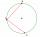There is a given circle k (center S, radius r). From point A which lies on circle k are starting two chords of length r. What angle does chords make? Draw and measure.
2. Circle r,DCalculate the diameter and radius of the circle if it has length 52.45 cm.
3. Plane IIA plane flew 50 km on a bearing 63degrees20 and the flew on a bearing 153degrees20 for 140km. Find the distance between the starting point and the ending point
4. Isosceles - isoscelesIt is given a triangle ABC with sides /AB/ = 3 cm /BC/ = 10 cm, and the angle ABC = 120°. Draw all points X such that true that BCX triangle is an isosceles and triangle ABX is isosceles with the base AB.
5. CableCable consists of 8 strands, each strand consists of 12 wires with diameter d = 0.5 mm. Calculate the cross-section of the cable.
6. Clock handsThe second hand has a length of 1.5 cm. How long does the endpoint of this hand travel in one day?
7. CircleWhat is the radius of the circle whose perimeter is 6 cm?
8. The bridge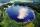Across the circle lakepasses through its center bridge over the lake. At three different locations on the lake shore are three fishermen A, B, C. Which of fishermen see the bridge under the largest angle?
9. DiagonalCan a rhombus have the same length diagonal and side?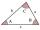The size of two internal angles of a triangle ABC are α=6/18π and β=7/18π. Calculate the size of the third angle.
11. Obtuse angleWhich obtuse angle is creating clocks at 17:00?
12. AnglesIn the triangle ABC, the ratio of angles is: a:b = 4: 5. The angle c is 36°. How big are the angles a, b?
13. 3-bracketMay be the largest angle in the triangle less than 20°?
14. Two anglesThe triangles ABC and A'B'C 'are similar. In the ABC triangle, the two angles are 25° and 65°. Explain why in the triangle A'B'C 'is the sum of two angles of 90 degrees.
15. Type of triangle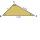How do I find the triangle type if the angle ratio is 2:3:7 ?
16. Angles in triangle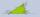Calculate the alpha angle in the triangle if beta is 61 degrees and 98 gamma degrees.
17. Triangle ABCConstruct a triangle ABC is is given c = 60mm hc = 40 mm and b = 48 mm analysis procedure steps construction